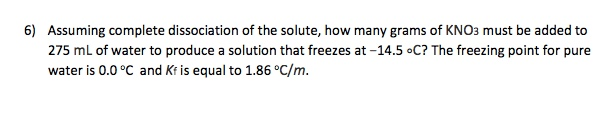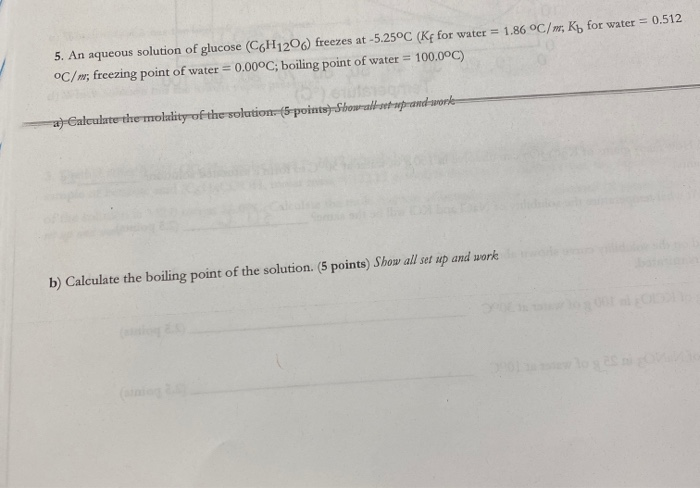Question

Question 1.

A 2.700×10−2M solution of NaCl in water is at 20.0∘C. The sample was created by dissolving a sample of NaCl in water and then bringing the volume up to 1.000 L. It was determined that the volume of water needed to do this was 999.3 mL . The density of water at 20.0∘C is 0.9982 g/mL.

a.)Calculate the molality of the salt solution.

B.) Calculate the mole fraction of salt in this solution.

C.) Calculate the concentration of the salt solution in percent by mass.

D.) Calculate the concentration of the salt solution in parts per million.

Question 2.

Assuming complete dissociation of the solute, how many grams of KNO3 must be added to 275 mL of water to produce a solution that freezes at −14.5 ∘C? The freezing point for pure water is 0.0 ∘C and Kf is equal to 1.86 ∘C/m.

Molarity = mole of solute/mass of solvent

mole of solute= molarity of solution/volume of solution

. = 0.0205M × 1.000L

= 0.02050

#### Earn Coins

Coins can be redeemed for fabulous gifts.

Similar Homework Help Questions
• ### A 2.550×10−2M solution of NaCl in water is at 20.0∘C. The sample was created by dissolving...

A 2.550×10−2M solution of NaCl in water is at 20.0∘C. The sample was created by dissolving a sample of NaCl in water and then bringing the volume up to 1.000 L. It was determined that the volume of water needed to do this was 999.3 mL . The density of water at 20.0∘C is 0.9982 g/mL. A) Calculate the molality of the salt solution. B) Calculate the mole fraction of salt in this solution. C) Calculate the concentration of the...

• ### A 2.400×10−2 M solution of NaCl in water is at 20.0∘C. The sample was created by...

A 2.400×10−2 M solution of NaCl in water is at 20.0∘C. The sample was created by dissolving a sample of NaCl in water and then bringing the volume up to 1.000 L. It was determined that the volume of water needed to do this was 999.4 mL . The density of water at 20.0∘C is 0.9982 g/mL. a. Calculate the molality of the salt solution. b. Calculate the mole fraction of salt in this solution. c. Calculate the concentration of...

• ### A) Calculate the osmotic pressure of a solution containing 16.75 mg of hemoglobin in 15.7 mL...

A) Calculate the osmotic pressure of a solution containing 16.75 mg of hemoglobin in 15.7 mL of solution at 22 ∘C . The molar mass of hemoglobin is 6.5×104 g/mol. B) A 2.400×10−2M solution of NaCl in water is at 20.0∘C. The sample was created by dissolving a sample of NaCl in water and then bringing the volume up to 1.000 L. It was determined that the volume of water needed to do this was 999.4 mL . The density...

• ### 6) Assuming complete dissociation of the solute, how many grams of KNO3 must be added to...6) Assuming complete dissociation of the solute, how many grams of KNO3 must be added to 275 mL of water to produce a solution that freezes at -14.5 °C? The freezing point for pure water is 0.0°C and Kf is equal to 1.86 °C/m.

• ### Chemists often use molarity M, in moles/liter, to measure the concentration of solutions. Molarity is a...

Chemists often use molarity M, in moles/liter, to measure the concentration of solutions. Molarity is a common unit of concentration because the volume of a liquid is very easy to measure. However, the drawback of using molarity is that volume is a temperature-dependent quantity. As temperature changes, density changes, which affects volume. Volume markings for most laboratory glassware are calibrated for room temperature, about 20∘C. A 2.500×10−2M solution of NaCl in water is at 20.0∘C. The sample was created by...

• ### What mass of salt (NaCl) should you add to 1.14 L of water in an ice-cream...

What mass of salt (NaCl) should you add to 1.14 L of water in an ice-cream maker to make a solution that freezes at -11.8 C? Assume complete dissociation of the NaCl and a density of 1.00 g/mL for water and use Kf=1.86 C/m.

• ### A salt solution was made by dissolving 17.32 g of NaCl in 400.0 mL of water....

A salt solution was made by dissolving 17.32 g of NaCl in 400.0 mL of water. Calculate the molality (NOT molarity) of this solution Hint: Molality (m) = moles of solute / Kg of solvent Use 11Na23 and 17Cl35.5

• ### Questions number 5 5. An aqueous solution of glucose (C6H1206) freezes at -5.25°C (Kf for water...Questions number 5 5. An aqueous solution of glucose (C6H1206) freezes at -5.25°C (Kf for water = 1.86 °C/n, Kb for water = 0.512 °C/n, freezing point of water = 0.00°C; boiling point of water = 100.0°C) a) Calculate the molality of the solution (S-points) Showu wrand worke b) Calculate the boiling point of the solution. (5 points) Show all set up and work !

• ### What is the freezing point of an aqueous 1.00 m NaCl solution? (Kf = 1.86 *C/m)...

What is the freezing point of an aqueous 1.00 m NaCl solution? (Kf = 1.86 *C/m) (Assume complete dissociation of the salt.) I know the answer is -3.78*C but I cannot figure out how to get it. I keep getting -1.86*C. Please show step by step so I can understand. Thank you.

• ### An aqueous CaCl2 solution has a vapor pressure of 80.2 mmHg at 50 ∘C. The vapor pressure of pure water at this temperature is 92.6 mmHg. What is the concentration of CaCl2 in mass percent? A hypotheti...

An aqueous CaCl2 solution has a vapor pressure of 80.2 mmHg at 50 ∘C. The vapor pressure of pure water at this temperature is 92.6 mmHg. What is the concentration of CaCl2 in mass percent? A hypothetical solution forms between a solid and a liquid. The values of the thermodynamic quantities involved in the process are shown in the following table. Action Enthalpy separation of solute 11.5 kJ/mol separation of solvent 21.8 kJ/mol formation of solute-solvent interactions -86.7 kJ/mol solute...